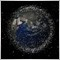How to pass a struct MqlRates member to a function as an array?138

Hello,

I 'm trying to figure out how to pass struct MqlRates members to a function  as arrays but the compiler refuses stubbornly coding like open, rt.open and rt[].open, maybe some sort of a pointer?

here is the relevant part of the code.

-----------------------------------------------------------------------------------------------------------------------------------------------------------------------------------------------------------------------------

int OnCalculate(const int rates_total,
const int prev_calculated,
const datetime &Time[],
const double &Open[],
const double &High[],
const double &Low[],
const double &Close[],
const long &TickVolume[],
const long &Volume[],

This compiles:

reBuild(rates_total, prev_calculated, open, high, Low, close, time);

----

MqlRates rt[];
if(CopyRates(NULL,InpTimeFrame,0,InpTimeBarsLimit,rt)!=InpTimeBarsLimit)
else

This does not compile:

reBuild(rates_total, prev_calculated, rt.open, rt.high, rt.Low, rt.close, rt.time);

----

void reBuild(const int rates_total, const int prev_calculated, const double &Open[], const double &High[], const double &Low[], const double &Close[], const datetime &Time[])

-----------------------------------------------------------------------------------------------------------------------------------------------------------------------------------------------------------------------------

Is there a way to pass MqlRates  members to a function?